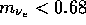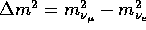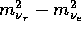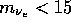Next: WIMPs Up: Neutrinos as Dark Previous: Neutrinos as Dark

## Detecting Massive Neutrinos

While it is very difficult to detect the cosmic background of neutrinos directly, there are several experimental approaches that might be able to measure the mass of the neutrino. As I noted earlier, the detection of a stable several eV neutrino would imply that neutrinos comprise a significant fraction of the mass of the universe.

The classical approach to measuring neutrino mass are measurements of thedecay endpoint. Current limits from these experiments imply that the electron neutrino is not the predominant component of the dark matter; however, these experiments cannot place astrophysically interesting constraints on the mass of the mu or tau neutrino.

If the neutrino is a Majorana particle, then it might be indirectly detected through the detection of a neutrinoless double beta decay. Deep underground experiments looking for rare decays have placed very interesting limits  on the electron neutrino mass:eV. This is a limit on massive neutrinos if the most massive eigenstate contains a significant fraction of the electron flavor eigenstate and does not apply to all neutro models.

Neutrino oscillation experiments are sensitive to mass differences, usuallyand sometime. Recent results from the Los Alamos experiment , which suggest a detection of neutrino oscillations, are controversial .

There is a possibility of an astronomical detection of neutrino mass using neutrinos from a supernova explosion. If the neutrinos are massive, then more-energetic neutrinos arrive earlier than less-energetic neutrinos. Thus, neutrino detectors would first see higher energy events and then see less energetic events. This effect was not observed in SN 1987A, which suggests thateV . Observations of a galactic supernova by Sudbury detector, which is sensitive tocould place interesting limits on their masses and possibly rule out neutrinos as cosmologically interesting.

Dave Spergel
Wed Mar 6 14:02:15 EST 1996